SPSS TUTORIALS BASICS ANOVA REGRESSION FACTOR CORRELATION

# SPSS Chi-Square Test with Pairwise Z-Tests

Most data analysts are familiar with post hoc tests for ANOVA. Oddly, post hoc tests for the chi-square independence test are not widely used. This tutorial walks you through 2 options for obtaining and interpreting them in SPSS.

## Example Data

A sample of N = 300 respondents were asked about their education level and marital status. The data thus obtained are in edu-marit.sav. All examples in this tutorial use this data file.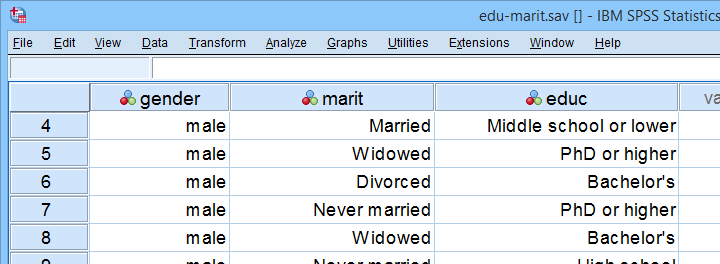## Chi-Square Independence Test

Right. So let's see if education level and marital status are associated in the first place: we'll run a chi-square independence test with the syntax below. This also creates a contingency table showing both frequencies and column percentages.

*Basic crosstabs with chi-square independence test.

crosstabs marit by educ
/cells count column
/statistics chisq.

Let's first take a look at the actual test results shown below.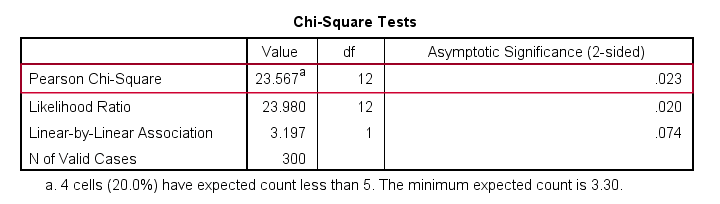First off, we reject the null hypothesis of independence: education level and marital status are associated, χ2(12) = 23.57, p = 0.023. Note that that SPSS wrongfully reports this 1-tailed significance as a 2-tailed significance. But anyway, what we really want to know is precisely which percentages differ significantly from each other?

## Option 1 - CROSSTABS

We'll answer this question by slightly modifying our syntax: adding BPROP (short for “Bonferroni proportions”) to the /CELLS subcommand does the trick.

*Crosstabs with pairwise z-tests.

crosstabs marit by educ
/cells count column bprop. /*bprop = Bonferroni adjusted z-tests for column proportions.

Running this simple syntax results in the table shown below.

## CROSSTABS with Pairwise Z-Tests Output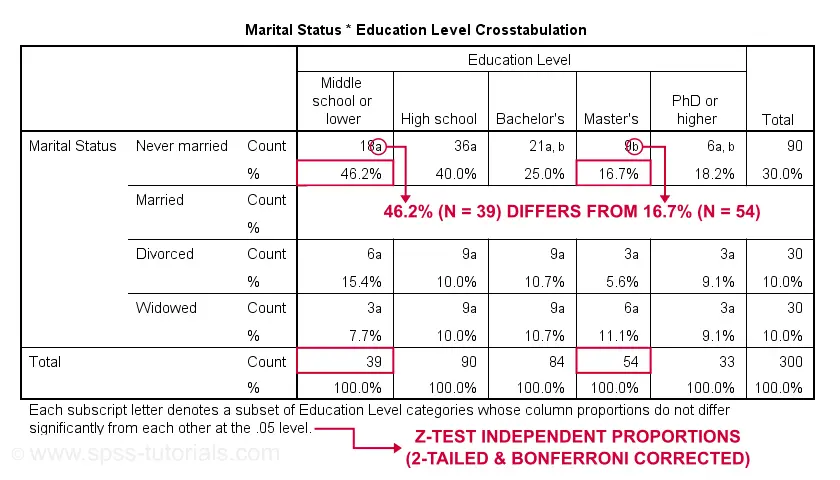First off, take a close look at the table footnote: “Each subscript letter denotes a subset of Education Level categories whose column proportions do not differ significantly from each other at the .05 level.”
These conclusions are based on z-tests for independent proportions. These also apply to the percentages shown in the table: within each row, each possible pair of percentages is compared using a z-test. If they don't differ, they get a similar subscript. Reversely, within each row, percentages that don't share a subscript
are significantly different.
For example, the percentage of people with middle school who never married is 46.2% and its frequency of n = 18 is labeled “a”. For those with a Master’s degree, 16.7% never married and its frequency of 9 is not labeled “a”. This means that 46.2% differs significantly from 16.7%.

The frequency of people with a Bachelor’s degree who never married (n = 21 or 25.0%) is labeled both “a” and “b”. It doesn't differ significantly from any cells labeled “a”, “b” or both. Which are all cells in this table row.

Now, a Bonferroni correction is applied for the number of tests within each row. This means that for $$k$$ columns,
$$P_{bonf} = P\cdot\frac{k(k - 1)}{2}$$
where

• $$P_{bonf}$$ denotes a Bonferroni corrected p-value and
• $$P$$ denotes a “normal” (uncorrected) p-value.

Right, now our table has 5 education levels as columns so $$P_{bonf} = P\cdot\frac{5(5 - 1)}{2} = P \cdot 10$$
which means that each p-value is multiplied by 10 and only then compared to alpha = 0.05. Or -reversely- only z-tests yielding an uncorrected p < 0.005 are labeled “significant”. This holds for all tests reported in this table. I'll verify these claims later on.

## Option 2 - Custom Tables

A second option for obtaining “post hoc tests” for chi-square tests are Custom Tables. They're found under AnalyzeTablesCustom Tables but only if you have a Custom Tables license. The figure below suggests some basic steps.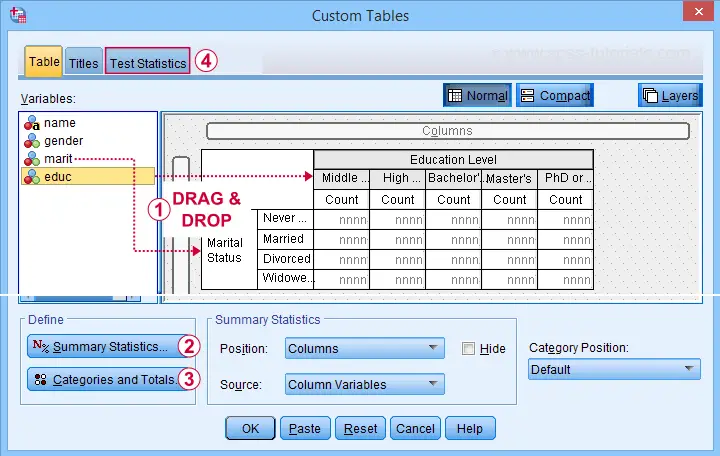You probably want to select both frequencies and column percentages for education level.We recommend you add totals for education levels as well.

Next, our z-tests are found in the Test Statistics tab shown below.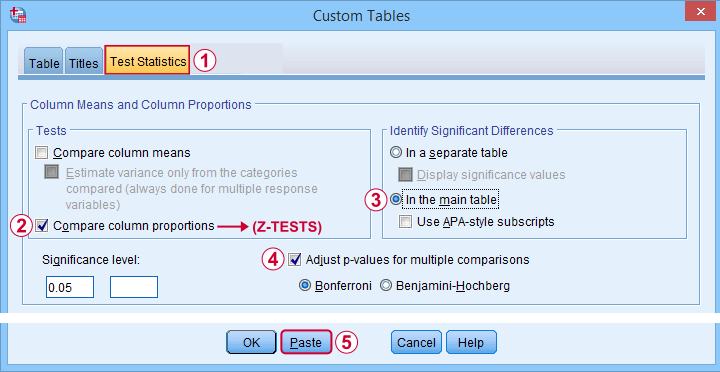Completing these steps results in the syntax below.

*Contingency table with pairwise z-tests via CTABLES.

CTABLES
/VLABELS VARIABLES=marit educ DISPLAY=DEFAULT
/TABLE marit BY educ [COUNT 'N' F40.0, COLPCT.COUNT '%' PCT40.1]
/CATEGORIES VARIABLES=marit ORDER=A KEY=VALUE EMPTY=INCLUDE TOTAL=YES POSITION=AFTER
/CATEGORIES VARIABLES=educ ORDER=A KEY=VALUE EMPTY=INCLUDE
/CRITERIA CILEVEL=95
/COMPARETEST TYPE=PROP ALPHA=0.05 ADJUST=BONFERRONI ORIGIN=COLUMN INCLUDEMRSETS=YES
CATEGORIES=ALLVISIBLE MERGE=YES STYLE=SIMPLE SHOWSIG=NO.

## Custom Tables with Pairwise Z-Tests Output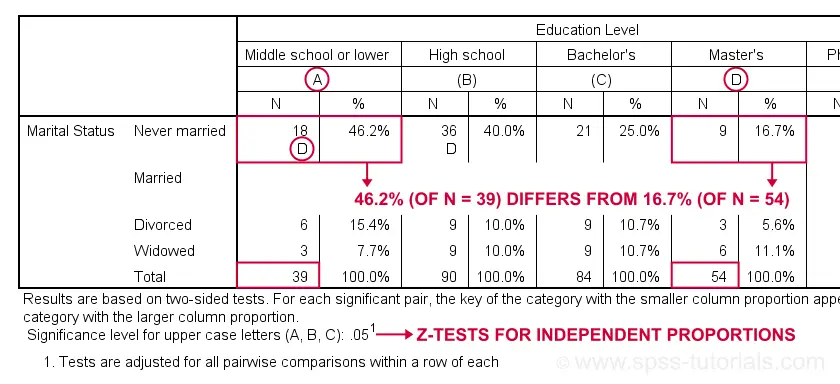Let's first try and understand what the footnote says: “Results are based on two-sided tests. For each significant pair, the key of the category with the smaller column proportion appears in the category with the larger column proportion. Significance level for upper case letters (A, B, C): .05. Tests are adjusted for all pairwise comparisons within a row of each innermost subtable using the Bonferroni correction.”

Now, for normal 2-way contingency tables, the “innermost subtable” is simply the entire table. Within each row, each possible pair of column proportions is compared using a z-test. If 2 proportions differ significantly, then the higher is flagged with the column letter of the lower. Somewhat confusingly, SPSS flags the frequencies instead of the percentages.

In the first row (never married), the D in column A indicates that these 2 percentages
differ significantly:
the percentage of people who never married is significantly higher for those who only completed middle school (46.2% from n = 39) than for those who completed a Master’s degree (16.7% from n = 54).

Again, all z-tests use α = 0.05 after Bonferroni correcting their p-values for the number of columns in the table. For our example table with 5 columns, each p-value is multiplied by $$0.5\cdot5(5 - 1) = 10$$ before evaluating if it's smaller than the chosen alpha level of 0.05.

## Can these Z-Tests be Replicated?

Yes. They can.

Custom Tables has an option to create a table containing the exact p-values for all pairwise z-tests. It's found in the Test Statistics tab. Selecting it results in the syntax below.

*Contingency table with Bonferroni corrected z-tests (exact p-values) in separate table.

CTABLES
/VLABELS VARIABLES=marit educ DISPLAY=DEFAULT
/TABLE marit BY educ [COUNT 'N' F40.0, COLPCT.COUNT '%' PCT40.1]
/CATEGORIES VARIABLES=marit ORDER=A KEY=VALUE EMPTY=INCLUDE TOTAL=YES POSITION=AFTER
/CATEGORIES VARIABLES=educ ORDER=A KEY=VALUE EMPTY=INCLUDE
/CRITERIA CILEVEL=95
/COMPARETEST TYPE=PROP ALPHA=0.05 ADJUST=BONFERRONI ORIGIN=COLUMN INCLUDEMRSETS=YES
CATEGORIES=ALLVISIBLE MERGE=NO STYLE=SIMPLE SHOWSIG=YES.

## Exact P-Values for Z-Tests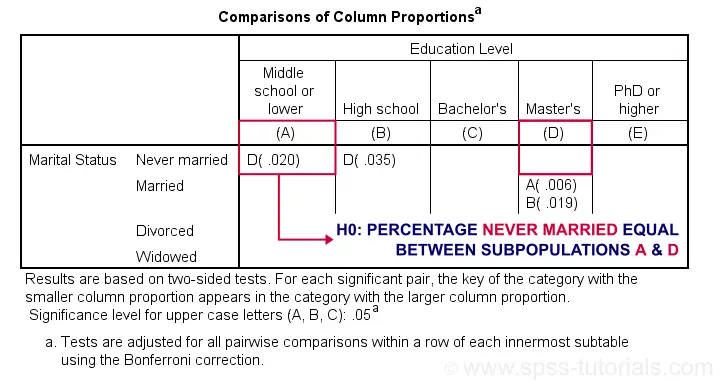For the first row (never married), SPSS claims that the Bonferroni corrected p-value for comparing column percentages A and D is p = 0.020. For our example table, this implies an uncorrected p-value of p = 0.0020.
We replicated this result with an Excel z-test calculator. Taking the Bonferroni correction into account, it comes up with the exact same p-value as SPSS.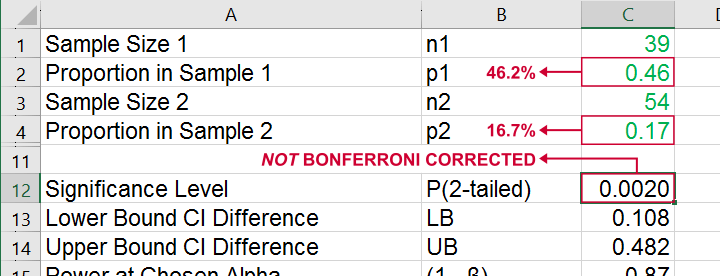All other p-values reported by SPSS were also exactly replicated by our Excel calculator.

I hope this tutorial has been helpful for obtaining and understanding pairwise z-tests for contingency tables. If you've any questions or feedback, please throw us a comment below.

# Tell us what you think!

*Required field. Your comment will show up after approval from a moderator.

# THIS TUTORIAL HAS 14 COMMENTS:

•### By Ruben Geert van den Berg on May 14th, 2021

Hi Jes!

Under Custom Tables -> Test Statistics you can select "Display significance values" (upper right half).

Hope that helps!

•### By Sittichai Charoen on June 19th, 2021

This method can call p-test ? Do you have detail about p-test ? Thank you for your reply to me in my e-mail.

•### By Sunjoo on September 22nd, 2021

I am need know how to organize the sample results table- [CROSSTABS with Pairwise Z-Tests Output] for research paper. I mean, in ANOVA we summarize the post hoc test results, for example, like ab ? thanks,

•### By Ruben Geert van den Berg on September 23rd, 2021

Hi Sunjoo!

Please look up SPSS Chi-Square Independence Test and scroll down to "Adjusting Our Table".

This is a very powerful technique that you surely want to know about. These operations can be carried with with a Python script as well if needed.

Other adjustments are best done with OUTPUT MODIFY and/or in Excel.

Hope that helps!

SPSS tutorials